Random Variables and Random Process

# Random Variables and Random Process - Electronics and Communication Engineering (ECE)

 1 Crore+ students have signed up on EduRev. Have you?

Two random variables

Thus far, we have considered single random variables. In many situations, however, there may be more than one quantity that we are interested in knowing during a random experiment. For instance, in an experiment where we flip a coin ten times, we may care about both X (ω) = the number of heads that come up as well as Y (ω) = the length of the longest run of consecutive heads. In this section, we consider the setting of two random variables.

3.1 Joint and marginal distributions

Suppose that we have two random variables X and Y . One way to work with these two random variables is to consider each of them separately. If we do that we will only need FX (x) and FY (y). But if we want to know about the values that X and Y assume simultaneously during outcomes of a random experiment, we require a more complicated structure known as the joint cumulative distribution function of X and Y , defined by

FXY(x, y) = P (X x, Y y)

It can be shown that by knowing the joint cumulative distribution function, the probability of any event involving X and Y can be calculated.

The joint CDF FX Y (x, y) and the joint distribution functions FX (x) and FY (y) of each variable separately are related by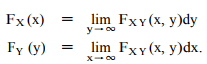∞

Here, we call FX (x) and FY (y) the marginal cumulative distribution functions of FX Y (x, y).

Properties: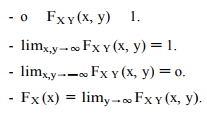3.2 Joint and marginal probability mass functions

If X and Y are discrete random variables, then the joint probability mass function pX Y : R×R → [o, 1] is defined by

pXY (x, y) = P (X = x, Y = y).

Here, o PXY (x, y) 1 for all x, y, and Px∈V al(X) Py∈V al(Y) PXY (x, y) = 1. How does the joint PMF over two variables relate to the probability mass function for each variable separately? It turns out that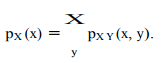and similarly for pY (y). In this case, we refer to pX (x) as the marginal probability mass function of X . In statistics, the process of forming the marginal distribution with respect to one variable by summing out the other variable is often known as ―marginalization.‖

3.3 Joint and marginal probability density functions

Let X and Y be two continuous random variables with joint distribution function FX Y . In the case that FX Y (x, y) is everywhere differentiable in both x and y, then we can

define the joint probability density function,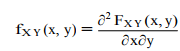Like in the single-dimensional case, fX Y (x, y) = P (X = x, Y = y), but rather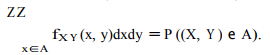Note that the values of the probability density function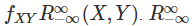are always nonnegative, but they may be greater than 1. Nonetheless, it must be the case that fXY (x,y) = 1. Analagous to the discrete case, we define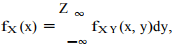as the marginal probability density function (or marginal density) of X , and similarly for fY (y).

3.4 Conditional distributions

Conditional distributions seek to answer the question, what is the probability distribution over Y , when we know that X must take on a certain value x? In the discrete case, the conditional probability mass function of X given Y is simply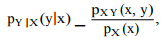assuming that pX (x) _ o. pY |X(y|x) _ p (x) , In the continuous case, the situation is technically a little more complicated because the probability that a continuous random variable X takes on a specific value x is equal to zero4 . Ignoring this technical point, we simply define, by analogy to the discrete case, the conditional probability density of Y given Xx to be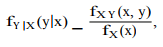provided fX (x) _ o.

3.5 Bayes‘s rule

A useful formula that often arises when trying to derive expression for the conditional probability of one variable given another, is Bayes‘s rule.

In the case of discrete random variables X and Y ,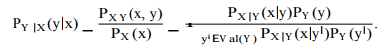If the random variables X and Y are continuous,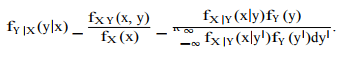3.6 Independence

Two random variables X and Y are independent if FXY (x, y) _ FX (x)FY(y) for all values of x and y. Equivalently,

• For discrete random variables, pXY(x, y) _ pX (x)pY (y) for all x e V al(X ), y e V al(Y ).
• For discrete random variables, pY |X(y|x) _ pY (y) whenever pX (x) _ o for all y e V al(Y ).
• For continuous random variables, fXY (x, y) _ fX (x)fY (y) for all x, y e R.
• For continuous random variables, fY |X(y|x) _ fY (y) whenever fX (x) _ o for all y e R.

To get around this, a more reasonable way to calculate the conditional CDF is,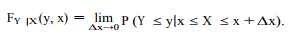It can be easily seen that if F (x, y) is differentiable in both x, y then,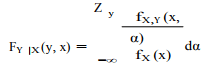and therefore we define the conditional PDF of Y given X = x in the following way,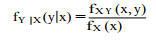Informally, two random variables X and Y are independent if ―knowing‖ the value of one variable will never have any effect on the conditional probability distribution of the other variable, that is, you know all the information about the pair (X, Y ) by just knowing f(x) and f(y). The following lemma formalizes this observation:

Lemma 3.1.

If X and Y are independent then for any subsets A, B ⊆ R, we have P (X e A, y e B) _ P (X e A)P (Y e B)

By using the above lemma one can prove that if X is independent of Y then any function of X is independent of any function of Y .

3.7 Expectation and covariance

Suppose that we have two discrete random variables X, Y and g : R -→ R is a function of these two random variables. Then the expected value of g is defined in the following way,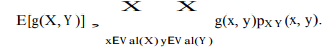For continuous random variables X, Y , the analogous expression is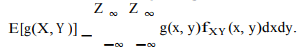We can use the concept of expectation to study the relationship of two random variables with each other. In particular, the covariance of two random variables X and Y is defined as

Cov[X, Y ] , E[(X - E[X])(Y - E[Y ])]

Using an argument similar to that for variance, we can rewrite this as,

Cov[X, Y ] _ E[(X - E[X])(Y - E[Y ])]
_ E[XY - X E[Y ] - Y E[X] + E[X]E[Y ]]
_ E[XY ] - E[X]E[Y ] - E[Y ]E[X] + E[X]E[Y ]]
_ E[XY ] - E[X]E[Y ].

Here, the key step in showing the equality of the two forms of covariance is in the third equality, where we use the fact that E[X] and E[Y ] are actually constants which can be pulled out of the expectation. When Cov[X, Y ] _ o, we say that X and Y are uncorrelated5 .

Properties:

- (Linearity of expectation) E[f(X, Y ) + g(X, Y )] _ E[f(X, Y )] + E[g(X, Y )].
- V ar[X + Y ] _ V ar[X] + V ar[Y ] + 2Cov[X, Y ].
- If X and Y are independent, then Cov[X, Y ] _ o.
- If X and Y are independent, then E[f(X )g(Y )] _ E[f(X )]E[g(Y )].

4 Multiple random variables

The notions and ideas introduced in the previous section can be generalized to more than two random variables. In particular, suppose that we have n continuous random variables, X1 (ω), X2 (ω), . . . Xn (ω). In this section, for simplicity of presentation, we focus only on the continuous case, but the generalization to discrete random variables works similarly.

4.1 Basic properties

We can define the joint distribution function of X1 , X2 , . . . , Xn , the joint probability density function of X1 , X2 , . . . , Xn , the marginal probability density function of X1 \, and the conditional probability density function of X1given X2 , . . . , Xn , as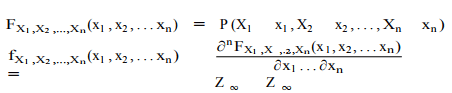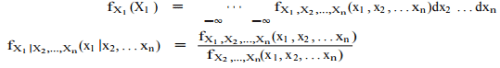To calculate the probability of an event A ⊆ R n we have,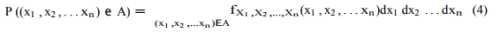Chain rule: From the definition of conditional probabilities for multiple random variables, one can show that

f(x1 , x2 , . . . , xn ) = f(xn |x1, x2 . . . , xn—1)f(x1, x2 . . . , xn—1)
= f(xn |x1, x2 . . . , xn—1)f(xn—1|x1, x2 . . . , xn—2)f(x1 , x2 . . . , xn—2)

= . . . = f(x1 ) Y f(xi|x1, . . . , xi—1).

i=2

Independence: For multiple events, A1 , . . . , Ak , we say that A1 , . . . , Ak are mutually independent if for any subset S ⊆ {1, 2, . . . , k}, we have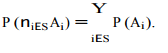Likewise, we say that random variables X1 , . . . , Xn are independent if

f(x1 , . . . , xn ) = f(x1 )f(x2 ) · · · f(xn )

Here, the definition of mutual independence is simply the natural generalization of independence of two random variables to multiple random variables.

Independent random variables arise often in machine learning algorithms where we assume that the training examples belonging to the training set represent independent samples from some unknown probability distribution. To make the significance of independence clear, consider a ―bad‖ training set in which we firstsample a single training example (x (1) , y (1) ) from the some unknown distribution, and then add m - 1 copies of the exact same training example to the training set. In this case, we have (with some abuse of notation)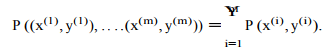Despite the fact that the training set has size m, the examples are not independent! While clearly the procedure described here is not a sensible method for building a training set for a machine learning algorithm, it turns out that in practice, non-independence of samples does come up often, and it has the effect of reducing the ―effective size‖ of the training set.

Random vectors

Suppose that we have fl random variables. When working with all these random variables together, we will often find it convenient to put them in a vector X = [X1 X2 . . . Xn ] T . We call the resulting vector a random vector (more formally, a random vector is a mapping from Ito Rn ). It should be clear that random vectors are simply an alternative notation for dealing with fl random variables, so the notions of joint PDF and CDF will apply to random vectors as well. Expectation: Consider an arbitrary function from g : Rn → R. The expected value of this function is defined as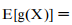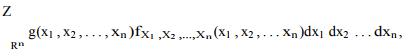where R Rn is fl consecutive integrations from -∞ to ∞. If g is a function from Rn to Rm , then the expected value of g is the element-wise expected values of the output vector, i.e., if g is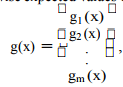Then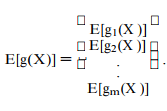Covariance matrix: For a given random vector X : I → R n , its covariance matrix Σ is the fl × fl square matrix whose entries are given by Σij = Cov[Xi , Xj ]. From the definition of covariance, we have

From the definition of covariance, we have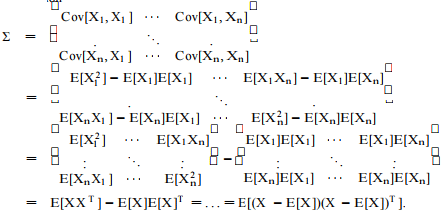here the matrix expectation is defined in the obvious way.
The covariance matrix has a number of useful

properties:

- Σ 0; that is, Σ is positive semi definite.
- Σ = Σ T ; that is, Σ is symmetric.

4.3 The multivariate Gaussian distribution

One particularly important example of a probability distribution over random vectors X is called the multivariate Gaussian or multivariate normal distribution. A random vector X e Rn is said to have a multivariate normal (or Gaussian) distribution with mean µ e Rn and covariance matrix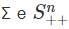(where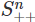refers to the space of symmetric positive definite fl × fl matrices)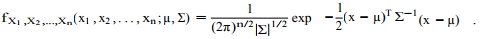We write this as X ∼ N (µ, Σ). Notice that in the case fl = 1, this reduces the regular definition of a normal distribution with mean parameter µ1 and variance Σ11 . Generally speaking, Gaussian random variables are extremely useful in machine learning and statistics for two main reasons. First, they are extremely common when modeling ―noise‖ in statistical algorithms. Quite often, noise can be considered to be the accumulation of a large number of small independent random perturbations affecting the measurement process; by the Central Limit Theorem, summations of independent random variables will tend to ―look Gaussian.‖ Second, Gaussian random variables are convenient for many analytical manipulations, because many of the integrals involving Gaussian distributions that arise in practice have simple closed form solutions.

The document Random Variables and Random Process - Electronics and Communication Engineering (ECE) is a part of Electronics and Communication Engineering (ECE) category.
All you need of Electronics and Communication Engineering (ECE) at this link: Electronics and Communication Engineering (ECE)

Track your progress, build streaks, highlight & save important lessons and more!(Scan QR code)

,

,

,

,

,

,

,

,

,

,

,

,

,

,

,

,

,

,

,

,

,

;